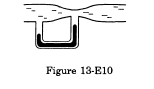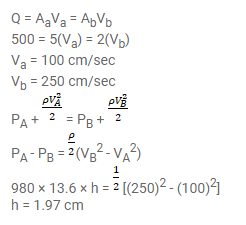# Water flows through the tube shown in figure(13-E10).`
Question:

Water flows through the tube shown in figure(13-E10). The areas of cross-section of the wide and the narrow portions of the tube are $5 \mathrm{~cm}^{2}$ and $2 \mathrm{~cm}^{2}$ respectively. The rate of flow of water through the tube is $500 \mathrm{~cm}^{3} / \mathrm{s}$. Find the difference of mercury levels in the U-tube.Solution: